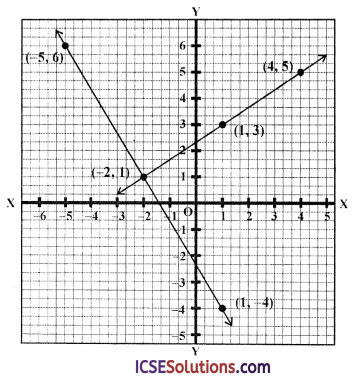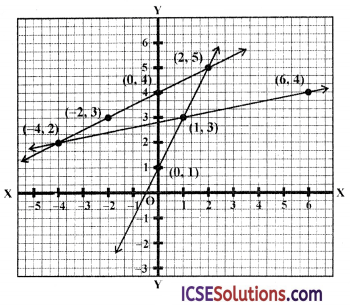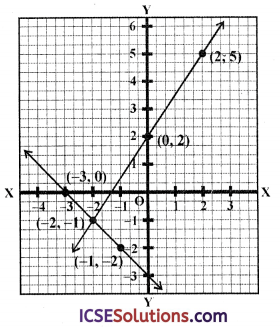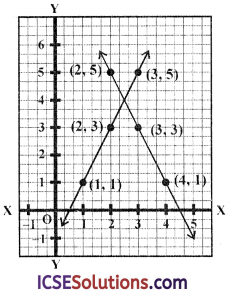Parents can use ICSE S Chand Maths Class 9 Solutions Chapter 20 Coordinates and Graphs of Simultaneous Linear Equations Ex 20(C) to provide additional support to their children.

## S Chand Class 9 ICSE Maths Solutions Chapter 20 Coordinates and Graphs of Simultaneous Linear Equations Ex 20(C)

Question 1.
3y – 2x = 7, 5x + 3y = -7
Solution:
3y – 2x = 7
⇒ 3y = 7 + 2x
⇒ y = $$\frac{7+2 x}{3}$$
Giving some different values to x, we get corresponding values of y as given below

 X 1 4 -2 y 3 5 1

Now plot the points (1, 3),(4, 5) and (-2, 1) on the graph and join them to get a line Similarly in equation,
5x + 3y = -7
⇒ 5x = -7 – 3y
x = $$\frac{-7-3 y}{5}$$
= $$-\left(\frac{7+3 y}{5}\right)$$

 X -2 -5 1 y 1 6 -4

Now plot the points (-2, 1), (-5, 6) and (1, -4) on the graph and join them to get another line. We see that these two lines intersect each other at (-2, 1)
∴ x = -2. y = 1Question 2.
2x + 3y = 13, 5x – 2y = 4
Solution:
2x + 3y = 13 ⇒ 2x = 13 – 3y
⇒ x = $$\frac{13-3 y}{2}$$
Giving some different values to y, we get corresponding values of x as given below

 X 5 2 -1 y 1 3 5

Now plot the points (5, 1), (2, 3) and (-1, 5) on the graph and join them to get a line similarly in equation
5x – 2y = 4
⇒ 5x = 4 + 2y
⇒ x = $$\frac{4+2 y}{5}$$

 X 2 0 -2 y 3 -2 -7

Now plot the points (2, 3), (0, -2) and (-2, -7) on the graph and join them to get another line
We see that these two lines intersect each other at the point (2, 3)
∴ x = 2, y = 3Question 3.
5x + y = -3, 2x = 3y – 8
Solution:
5x + y = -3 ⇒ y = -3 – 5x
⇒ y = -(3 + 5x)
Now giving some different values to x, we get the corresponding values of y as given below:

 X 0 -1 -2 y -3 2 7

Now plot the points (0, -3), (-1, 2) and (-2, 7) on the graph and join them to get a line Similarly in equation 2x = 4y – 8
⇒ x = $$\frac{3 y-8}{2}$$

 X -4 -1 2 y 0 2 4

Now plot the points (-4, 0), (-1, 2) and (2, 4) on the graph and join them to get another line
We see that these two lines intersect each other at (-1, 2)
∴ x = -1, y = 2Question 4.
Find graphically the vertices of the triangle whose sides have the equations 2y – x = 8, 5y – x = 14 and y – 2x = 1 respectively. Take 1 cm = 1 unit on both the axes.
Solution:
2y – x = 8, 5y – x = 14 and y – 2x = 1
Now 2y = 8 + x
y = $$\frac{8+x}{2}$$
Giving some different values to x, we get corresponding values of y as givne below :

 X 0 -2 -4 y 4 3 2

Now plot the points (0, 4), (-2, 3) and (-4, 2) on the graph and join them to get a line Similarly in equaiton,
5y – x = 14 ⇒ x = 5y – 14

 X -4 1 6 y 2 3 4

and join them to get another line and in equaiton
y – 2x = 1 => y = 1 + 2x

 X 0 1 2 y 1 3 5

Plot the points (0, 1), (1, 3) and (2, 5) on the graph and join them to get third lineWe see that the lines intersect each other as the points (-4, 2), (2, 5) and (1,3)
∴ The vertices of the triangle so formed are (-4, 2), (2, 5) and (1, 3)

Question 5.
Using a scale of 1 cm to 1 unit for both the axes, draw the graphs of the following equations :
6y = 5x + 10, y = 5x – 15 From the graphs find :
(i) the coordinates of the point where the two lines intersect ;
(ii) the area of the triangle between the lines and the x-axis.
Solution:
6y = 5x+ 10, y = 5x – 15
6y = 5x + 10
y = $$\frac{5 x+10}{6}$$
Giving some different values to x, we get corresponding values of y as given below :

 X 4 10 -2 y 5 10 0

Now plot the points (4, 5), (10, 10) and (-2, 0) on the graph and join them to get a line
Similarly in the equation,
y = 5x – 15

 X 2 3 4 y -5 0 5

Now plot the points (2, -5), (3, 0) and (4, 5) on the graph and join them to get another line(i) We see that these two lines intersect each other at the point (4, 5)
(ii) ∵ x-axis and the two lines form a triangle ABC whose base AC = 5 units and height = 5
∴ Area = $$\frac { 1 }{ 2 }$$ × 5 × 5 = $$\frac { 25 }{ 2 }$$ = 12.5 sq. units
or 12.5 cm2

Question 6.
The graph of a linear equation in x and y
Solution:
x + y + 3 = 0, and 3x – 2y + 4 = 0
(i) In the equation
x + y + 3 = 0 ⇒ x = -(y + 3)
Given three different values to y, we get the corresponding values of x as shown below :

 X -3 -2 -1 y 0 -1 -2

Now plot these points (-3, 0), (-2, -1) and (-1, -2) on the graph and join them to get a line
Similarly in the equation,
3x – 2y + 4 = 0
⇒ 3x = 2y – 4
⇒ x = $$\frac{2 y-4}{3}$$
Now plot these points (-2, -1), (0, 2) and (2, 5) on the graph and join them to get another line(ii) We see that these two lines intersect each other at the point P (-2, -1)
∴ Co-ordinates of P are (-2, -1)

(iii) Join OP and on measuring OP,
We get OP = 2.2 (approx)

Question 9.
Solve graphically the simultaneous equations,
x – 2y = 1; x + y = 4.
Use 2 cm = 1 unit on both axes and plot only three points per line.
Solution:
The equations are
x – 2y = 1 and x + y – 4
In the equation x – 2y= 1
⇒ x = 1 + 2y
Giving three different values toy, we get the corresponding values of x as given below :

 X 1 3 5 y 0 1 2

Now plot the points (1, 0), (3, 1), (5, 2) on the graph and join them to get a line Similarly in the equation
x + y = 4 ⇒ x = 4 – y

 X 4 3 2 y 0 1 2

Now plot these points (4, 0) (3, 1) and (2, 2) on the graph and join them to get another line
We see that these two lines intersect each other at (3, 1)
∴ Solution is x = 3, y = 1Question 10.
Use a graph paper for this question. Draw graph of 2x – y – 1 =0 and 2x + y = 9 on the same axes. Use 2 cm = 1 unit on both axes and plot only 3 points for each line. Write down the coordinates of the point of intersection of the two lines.
Solution:
The two equations are 2x – y – 1 – 0 and 2x + y = 9
Now in the equations,
2x – y – 1 = 0
2x = y + 1
x = $$\frac{y+1}{2}$$
Now giving three different values to y, we get the corresponding values of x as given below:

 X 1 2 3 y 1 3 5

Now plot there points (1, 2), (2, 3) and (3, 5) on the graph and join them to get a line
Similarly in the equation,
2x + y = 9 ⇒ y = 9 – 2x

 X 2 3 4 y 5 3 1

Now plot these points (2, 5), (3, 3) and (4, 1) on the graph and join them to get another line
We see that these two lines intersect each other at (2.5, 4)